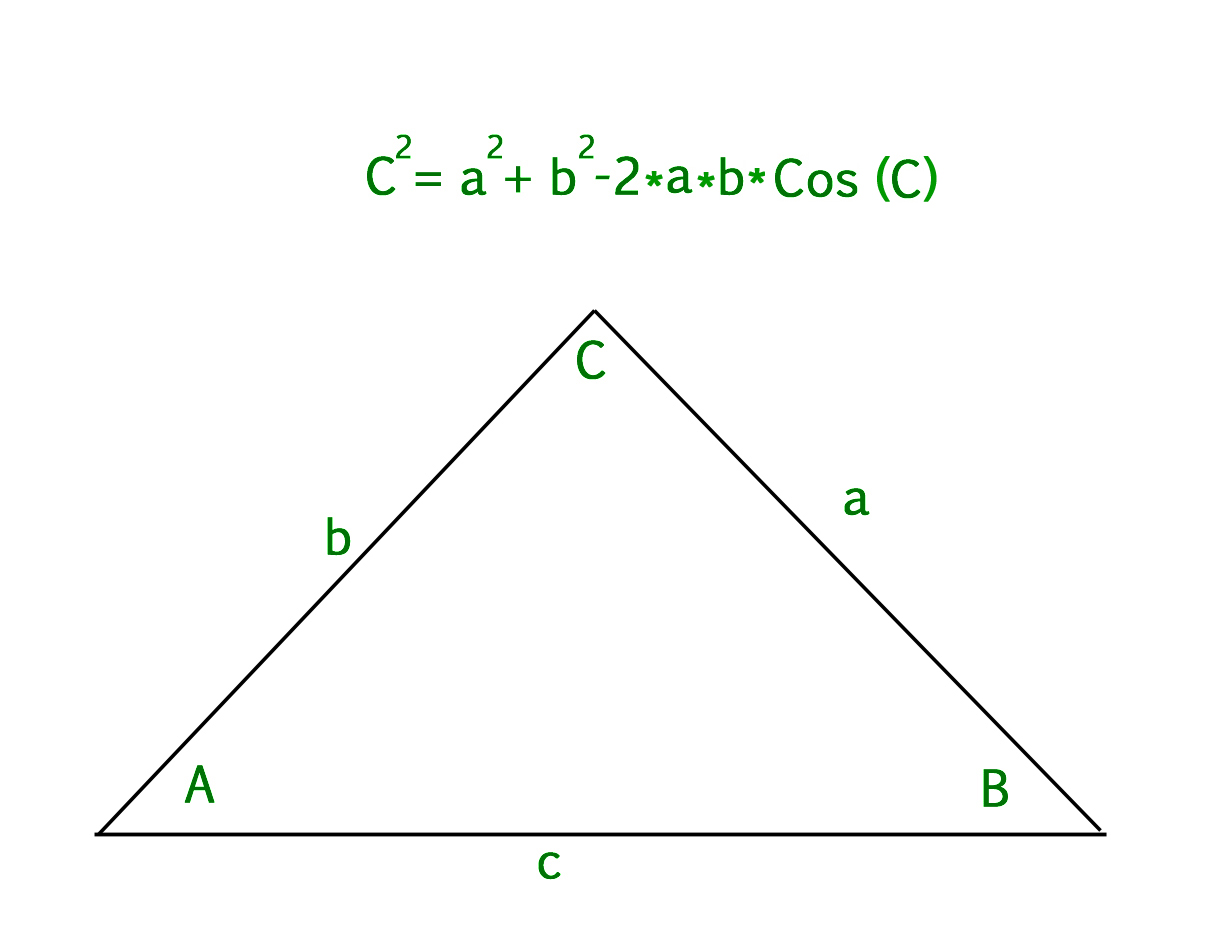# Program to find third side of triangle using law of cosines

• Difficulty Level : Medium
• Last Updated : 28 Jul, 2022

Given two sides A, B and angle C. Find the third side of the triangle using law of cosines.
Examples:

```Input : a = 5, b = 8, c = 49
Output : 6.04339 ```

In particular, the Law of Cosines can be used to find the length of the third side of a triangle when you know the length of two sides and the angle in between. See here to learn to how to find the value of cos.
Let us assume a, b, c are the sides of triangle where c is the side across from angle C. Then,

```c^2 = a^2 + b^2 - 2*a*b*cos(c)
OR
c = sqrt(a^2 + b^2 - 2*a*b*cos(c))```## C++

 `// CPP program to find third``// side of triangle using``// law of cosines` `#include ``using` `namespace` `std;` `// Function to calculate cos value of angle c``float` `cal_cos(``float` `n)``{``    ``float` `accuracy = 0.0001, x1, denominator, cosx, cosval;` `    ``// Converting degrees to radian``    ``n = n * (3.142 / 180.0);` `    ``x1 = 1;` `    ``// Maps the sum along the series``    ``cosx = x1;` `    ``// Holds the actual value of sin(n)``    ``cosval = ``cos``(n);``    ``int` `i = 1;``    ``do` `{``        ``denominator = 2 * i * (2 * i - 1);``        ``x1 = -x1 * n * n / denominator;``        ``cosx = cosx + x1;``        ``i = i + 1;``    ``} ``while` `(accuracy <= ``fabs``(cosval - cosx));` `    ``return` `cosx;``}` `// Function to find third side``float` `third_side(``int` `a, ``int` `b, ``float` `c)``{``    ``float` `angle = cal_cos(c);``    ``return` `sqrt``((a * a) + (b * b) - 2 * a * b * angle);``}``// Driver program to check the above function``int` `main()``{``    ``float` `c = 49;``    ``int` `a = 5, b = 8;``    ``// function call``    ``cout << third_side(a, b, c);` `    ``return` `0;``}`

## Java

 `// Java program to find third``// side of triangle using``// law of cosines``class` `GFG``{``    ``// Function to calculate``    ``// cos value of angle c``    ``static` `float` `cal_cos(``float` `n)``    ``{``        ``float` `accuracy = ``0``.0001f, x1;``        ``float` `denominator, cosx, cosval;``    ` `        ``// Converting degrees to radian``        ``n = n * (``3``.142f / ``180``.0f);``    ` `        ``x1 = ``1``;``    ` `        ``// Maps the sum along the series``        ``cosx = x1;``    ` `        ``// Holds the actual value of sin(n)``        ``cosval = (``float``)Math.cos(n);``        ``int` `i = ``1``;``        ``do` `{``            ``denominator = ``2` `* i * (``2` `* i - ``1``);``            ``x1 = -x1 * n * n / denominator;``            ``cosx = cosx + x1;``            ``i = i + ``1``;``        ``} ``while` `(accuracy <=``               ``Math.abs(cosval - cosx));``    ` `        ``return` `cosx;``    ``}``    ` `    ``// Function to find third side``    ``static` `float` `third_side(``int` `a,``                    ``int` `b, ``float` `c)``    ``{``        ``float` `angle = cal_cos(c);``        ` `        ``return` `(``float``)Math.sqrt((a * a) +``            ``(b * b) - ``2` `* a * b * angle);``}` `// Driver code``public` `static` `void` `main (String[] args)``{``    ``float` `c = ``49``;``    ``int` `a = ``5``, b = ``8``;``    ` `    ``// function call``    ``System.out.print(Math.round(third_side(a,``                    ``b, c)*``100000.0``)/``100000.0``);``}``}` `// This code is contributed by Anant Agarwal.`

## Python3

 `# Python3 program to find third side``# of triangle using law of cosines``import` `math as mt` `# Function to calculate cos``# value of angle c``def` `cal_cos(n):` `    ``accuracy ``=` `0.0001``    ``x1, denominator, cosx, cosval ``=` `0``, ``0``, ``0``, ``0` `    ``# Converting degrees to radian``    ``n ``=` `n ``*` `(``3.142` `/` `180.0``)` `    ``x1 ``=` `1` `    ``# Maps the sum along the series``    ``cosx ``=` `x1` `    ``# Holds the actual value of sin(n)``    ``cosval ``=` `mt.cos(n)``    ``i ``=` `1``    ``while` `(accuracy <``=` `abs``(cosval ``-` `cosx)):` `        ``denominator ``=` `2` `*` `i ``*` `(``2` `*` `i ``-` `1``)``        ``x1 ``=` `-``x1 ``*` `n ``*` `n ``/` `denominator``        ``cosx ``=` `cosx ``+` `x1``        ``i ``=` `i ``+` `1` `    ``return` `cosx` `# Function to find third side``def` `third_side(a, b, c):``    ``angle ``=` `cal_cos(c)``    ``return` `mt.sqrt((a ``*` `a) ``+``                   ``(b ``*` `b) ``-` `2` `*` `a ``*` `b ``*` `angle)` `# Driver Code``c ``=` `49``a, b ``=` `5``, ``8``print``(third_side(a, b, c))` `# This code is contributed by mohit kumar`

## C#

 `// C# program to find third``// side of triangle using``// law of cosines``using` `System;` `class` `GFG``{``    ``// Function to calculate``    ``// cos value of angle c``    ``static` `float` `cal_cos(``float` `n)``    ``{``        ``float` `accuracy = 0.0001f, x1;``        ``float` `denominator, cosx, cosval;``    ` `        ``// Converting degrees to radian``        ``n = n * (3.142f / 180.0f);``    ` `        ``x1 = 1;``    ` `        ``// Maps the sum along the series``        ``cosx = x1;``    ` `        ``// Holds the actual value of sin(n)``        ``cosval = (``float``)Math.Cos(n);``        ``int` `i = 1;``        ``do` `{``            ``denominator = 2 * i * (2 * i - 1);``            ``x1 = -x1 * n * n / denominator;``            ``cosx = cosx + x1;``            ``i = i + 1;``        ``} ``while` `(accuracy <=``                ``Math.Abs(cosval - cosx));``    ` `        ``return` `cosx;``    ``}``    ` `    ``// Function to find third side``    ``static` `float` `third_side(``int` `a,``                    ``int` `b, ``float` `c)``    ``{``        ``float` `angle = cal_cos(c);``        ` `        ``return` `(``float``)Math.Sqrt((a * a) +``               ``(b * b) - 2 * a * b * angle);``    ``}` `    ``// Driver code``    ``public` `static` `void` `Main ()``    ``{``        ``float` `c = 49;``        ``int` `a = 5, b = 8;``        ` `        ``// function call``        ``Console.WriteLine(Math.Round(third_side(a,``                        ``b, c)*100000.0)/100000.0);``    ``}``}` `// This code is contributed by vt_m.`

## PHP

 ``

## Javascript

 ``

Output

`6.04339`

Time Complexity: O(log(n)), since using inbuilt sqrt function
Auxiliary Space: O(1), as we are not using any extra space.

My Personal Notes arrow_drop_up